# LAKHMIR SINGH AND MANJIT KAUR Solutions for Class 9 Chemistry Chapter 3 - Atoms and Molecules

Page / Exercise

## Chapter 3 - Atoms and Molecules 128

Solution 1

International Union of Pure and Applied Chemistry

Solution 2

(a) Law of conservation of mass - Antonie Lavoisier

(b) Law of constant proportions - Joseph Proust

Solution 3

(a) Law of conservation of mass.

(b) Law of constant proportions.

## Chapter 3 - Atoms and Molecules 129

Solution 4

John Dalton

Solution 5

Atoms can neither be created nor destroyed.

Solution 6

The elements consist of atoms having fixed mass, and that the number and kind of atoms of each element in a given compound is fixed.

Solution 7

Solution 8

Law of Conservation of mass and law of constant proportions.

Solution 9

Law of constant proportions.

Solution 10

Law of Conservation of mass.

Solution 11

Atoms are the building blocks of matter.

Solution 12

The size of an atom indicated by its radius which is called 'atomic radius'.

Solution 13

The radius of an atom is usually expressed in 'nanometers'.

Solution 14

1 nanometer = 10-9 m

Solution 15

'nm' represents nanometer.

Solution 16

Because they are very very small.

Solution 17

False ; it is Co

Solution 18

The molecular mass of a substance is the relative mass of its molecule as compared with the mass of a Carbon-12 atom taken as 12 units.

Solution 19

This means that a molecule of oxygen is 32 times heavier than 1/12 of a Carbon-12 atom.

Solution 20

(a) 1:8

(b) Conservation of mass

Solution 21

(a) Carbon is used as a standard for atomic mass scale.

(b) Atom with 6 neutrons and 6 protons in its nucleus so that its mass number is 12.

(c) Mass = 12 u

Solution 22

The major drawback of Dalton's atomic theory is that atoms were thought to be indivisible. But, it is not true since atoms are divisible.

Solution 23

No, the statement is not valid because atoms can be divided into subatomic particles called electrons, protons and neutrons.

Solution 24

Yes, 'THE SCANNING TUNNELLING MICROSCOPE' enables people to see atoms. This microscope can produce computer generated images of the surface of elements which show the individual atoms. The atoms show up as blurred images.

Solution 25

The symbol of element is the "first letter" or "first letter and another letter" of the English name or Latin name of the element.

For example, symbol of Hydrogen is "H" and symbol of Calcium is "Ca".

Solution 26

(a)Ca, Mg

(b)Cu, Hg

Solution 27

Hydrogen- H, Helium-He, Lithium-Li , Beryllium-Be, Boron-B

Solution 28

Sodium - Na

Potassium - K

Iron - Fe

Copper - Cu

Mercury - Hg

Silver - Ag

Solution 29

Hg - Mercury

Au - Gold

Ag - Silver

Sn - Tin

Solution 30

The number of atoms present in one molecule of an element is called atomicity of that element.

For example, atomicity of sodium is 1 and that of nitrogen is 2.

Solution 31

(a) Oxygen = 2

(b) Ozone = 3

(c) Neon = 1

(d) Sulphur = 8

(e) Phosphorus = 4

(f) Sodium = 1

Solution 32

A chemical formula represents the composition of a molecule of the substance in terms of the symbols of the elements present in the molecule. It is also known as molecular formula.

Chemical formula of element - H2 for hydrogen

Chemical formula of compound - H2O for water

Solution 33

(a). Water- H2O; Elements present are Hydrogen and Oxygen.

(b).Ammonia- NH3; Elements present are Nitrogen and Hydrogen.

(c).Methane-CH4; Elements present are Carbon and Hydrogen.

(d).Sulphur dioxide-SO2; Elements present are Sulphur and Oxygen.

(e).Ethanol-C2H5OH; Elements present are carbon, hydrogen and oxygen.

Solution 34

2N represents two separate atoms of nitrogen and N2 represents one molecule of nitrogen.

## Chapter 3 - Atoms and Molecules 130

Solution 35

(a) O- one atom of oxygen

(b) 2O- two separate atoms of oxygen

(c) O2-one molecule of oxygen

(d)3O2-three molecules of oxygen

Solution 36

H2 represents two atoms of hydrogen, one atom of sulphur and four atoms of oxygen.

Solution 37

(a) Oxygen gas occurs as a diatomic molecule in nature.

(b) Noble gases occur as monoatomic gases in nature.

Solution 38

2H represents two separate atoms of hydrogen and H2 represents one molecule of hydrogen.

Solution 39

(a) N - one atom of nitrogen

(b) 2N - two separate atoms of nitrogen

(c) N2 - one molecule of nitrogen

(d) 2N2 - two molecules of nitrogen

Solution 40

Significance of formula of a substance-

1. Formula represents the name of the substance.

2. Formula represents one molecule of a substance.

3. Formula gives the number of atoms of each element present in one molecule.

4. Formula also represents one mole of molecules of the substance.

Solution 41

Significance of the formula H2O-

1. H2O represents water.

2. It represents one molecule of water.

3. H2O also represents 6.022 x 1023 molecules of water.

4. It represents 18gm of water.

Solution 42

Molecular formula of glucose = C6H12O6

Molecular mass of glucose = (6xC)+(12xH)+(6xO) =72 + 12 + 96 = 180u.

Solution 43

(a).Molecular mass of Hydrogen (H2) = 2 x H = 2 x 1 u = 2 u

(b).Molecular mass of oxygen (O2) = 2 x O = 2 x 16 u = 32 u

(c).Molecular mass of chlorine (Cl2) = 2 x Cl = 2 x 35.5 = 71 u

(d).Molecular mass of Ammonia (NH3) = 1 x N + 3 x H = 14 + 3 = 17 u

(e).Molecular mass of carbon dioxide (CO2) = 1 x C + 2 x O = 12 + 32 = 44 u

Solution 44

(a). Molecular mass of methane (CH4) = 12 + 4 = 16 u

(b). Molecular mass of ethane (C2H6) = 2 x 12 + 6 x 1 = 30 u

(c). Molecular mass of ethane (C2H4) = 2 x 12 + 4 x 1 = 28 u

(d). Molecular mass of ethyne (C2H2) = 2 x 12 + 2 x 1 = 26 u

Solution 45

(a) Molecular mass of Methanol(CH3OH) =

1xC + 3xH + 1xO +1xH = (12+3+16+1)u = 32u

(b) Molecular mass of Ethanol (C2H5OH) = 2xC + 5xH + 1xO +1xH

= (24 + 5 + 16 + 1) = 46u

Solution 46

Molecular mass of ethanoic acid (CH3COOH)

= 1xC + 3xH + 1xC + 2xO +1xH = 12+3+12+32+1 = 60u

Solution 47

Molecular mass of Nitric acid (HNO3) = 1xH + 1xN + 3xO

= (1 + 14 +48) u = 63 u

Solution 48

Molecular mass of chloroform (CHCl3) = 1 x C + 1 x H + 3 x Cl

= (12 + 1 + 106.5)u = 119.5 u

Solution 49

Molecular mass of hydrogen bromide (HBr) = 1 x H + 1 x Br

= (1 + 80) u = 81u

Solution 50

(a).Molecular mass of hydrogen sulphide (H2S) = 2xH + 1xS

= (2+32) u = 34u

(b). Molecular mass of Carbon disulphide (CS2) = 1xC + 2xS = (12+2 x 32) u = 76 u

Solution 51

Law of conservation of mass by LAVOISIER states that: "Mass can neither be created nor be destroyed in a chemical reaction". So, in a chemical reaction, the total mass of reactants must be equal to the total mass of products.

For example: When calcium carbonate is heated, a chemical reaction takes place to form calcium oxide and calcium carbonate. If 100 gms of calcium carbonate is decomposed completely, then 56 gms of calcium oxide and 44 gms of carbon dioxide are formed.

In the above example: the total mass of products = 56 gms (CaO) + 44gms (CO2) = 100 gms.

As total mass of products is equal to the total mass of reactant so, the law of conservation of mass is satisfied.

Solution 52

Law of constant proportion given by PROUST states that "A chemical compound always consists of the same elements combined together in the same proportion by mass."

For example: If we decompose 100 gms of pure water by passing electricity through it, then 11 gms of hydrogen and 89 gms of oxygen are obtained. Now, if we repeat this experiment by taking pure water from different sources (like river, sea, well, etc.), the same masses of hydrogen and oxygen elements are obtained in each case. They are always combined together in the same constant proportion of 11:89 or 1:8 by mass. And this is the law of constant proportions.

Solution 53

[a]. Postulates of Dalton's atomic theory:

a)  All the matter is made up of very small particles called 'atoms'.

b)  Atoms cannot be divided.

c)  Atoms can neither be created nor be destroyed.

d)  Atoms are of various kinds. There are as many kinds of atoms as are elements.

e)   All the atoms of a given element are identical in every respect, having the same mass, size and chemical properties.

f) Atoms of different elements differ in mass, size and chemical properties.

g) The 'number' and 'kind' of atoms in a given compound is fixed.

h) During chemical combination, atoms of different elements combine in small whole numbers to form compounds.

i) Atoms of the same elements can combine in more than one ratio to form more than one compound.

[b]. The postulate "The elements consists of atoms and that atoms can neither be created nor destroyed" can be used to explain the law of conservation of mass.

[c]. The postulate "The elements consist of atoms having fixed mass, and that the number and kind of atoms of each element in a given compound is fixed" can be used to explain the law of constant proportions.

Solution 54

(a).Significance of symbol of element -

i. It represents name of the element.

ii. It represents one atom of the element.

iii. It represents a definite mass of the element.

iv. It represents one mole of atoms of the element.

For example - C represents one atom of the element Carbon. It also represents 12 gms of Carbon.

(b). Significance of symbol H -

i. It represents Hydrogen element.

ii. It represents one atom of Hydrogen element.

iii. It represents one mole of Hydrogen atoms.

iv. It represents 2 gms of Hydrogen.

Solution 55

a) An atom is the smallest particle of an element that can take part in a chemical reaction. They usually exist in combination with the atoms of same element or another element.

b) A molecule is an electrically neutral group of two or more atoms chemically bonded together.

For example- Ozone gas has three oxygen atoms combined together, so ozone exists in the form of O3 molecule.

c) The molecule of an element contains two or more similar atoms chemically bonded together.

For example- A molecule of hydrogen element consists of 2 hydrogen atoms combined together.

Whereas the molecule of compound contains two or more different type of atoms chemically bonded together.

For example- The molecule of hydrogen chloride(HCl) contains two different type of atoms, i.e. H and Cl.

Solution 56

(a) One atomic mass unit is defined as exactly one-twelfth the mass of an atom of carbon-12. Its symbol is 'u'.

(b) The atomic mass of an element is the relative mass of its atom as compared with the mass of a carbon-12 atom taken as 12 units.

(c) It means that one atom of oxygen is 16 times heavier than 1/12 of a C-12 atom.

## Chapter 3 - Atoms and Molecules 131

Solution 75

According to question-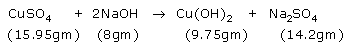Clearly, in this case

total mass of reactants = (15.95 gm + 8 gm) = 23.95 gm

total mass of products = (9.75 gm + 14.2 gm) = 23.95 gm

Hence, Law of conservation of mass is valid here.

Solution 77

According to question-

Reaction 1-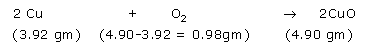,,So, 1 equivalent of Cu reacts with 0.25 equivalent of O2 to form 1.25 equivalent of copper oxide.

Reaction 2-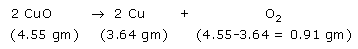Here again, one can see that 1.25 equivalent of CuO decomposed to form 1 equivalent of Cu and 0.25 equivalent of oxygen.

Hence, law of constant proportion is verified.

Solution 78

According to question-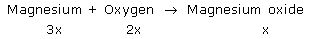i.e. three equivalents of Mg reacts with 2 equivalents of O2 to form 1 equivalent of MgO.

When mass of Mg = 3x = 24 gm

So, x = 8 gm

Then, mass of oxygen required = 2x = 16 gm

Solution
Solution
Solution
Solution
Solution

## Chapter 3 - Atoms and Molecules 132

Solution 79

When 5 gm of calcium is burnt in 2 gm of oxygen, then 7 gm of calcium oxide is formed. So, calcium and oxygen combine in the fixed proportion of 5:2 by mass.

Now, when 5 gm of calcium is burnt in 20 gm of oxygen, then also 7gm of calcium oxide will be formed because chemical reactions follows law of constant proportion.

As a result, 18 gm of oxygen will be left unreacted.

Solution 80

According to question-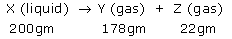(a) Liquid X - Water

Gas Y - Oxygen

Gas Z - Hydrogen

(b) mass of Z / mass of Y = 22gm / 178 gm = 1:8

(c) Law of constant proportion is illustrated by this example.

(d) Two sources of liquid X - Sea, Well

(e) Gas Y (oxygen) is necessary for breathing.

Solution 81

According to question-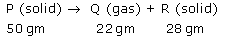(a) Solid P - Calcium Carbonate (CaCO3)

Gas Q - Carbon dioxide (CO2)

Solid R - Calcium oxide (CaO)

(b) Total mass of Q and R = 22gm + 28gm = 50gm

(c) Total mass of Q and R (50gm) is equal to mass of reactant (50gm).

(d) The law of conservation of mass is followed, i.e. total mass of product is equal to mass of reactant.

(e) Law of conservation of mass is illustrated by the example.

## Chapter 3 - Atoms and Molecules 150

Solution 1

Ions

Solution 2

(a). Anions ; (b). Cations

Solution 3

The formula mass of an ionic compound is the relative mass of its 'formula unit' as compared with the mass of a Carbon-12 atom taken as 12 units.

## Chapter 3 - Atoms and Molecules 151

Solution 4

(a) Anions are formed by the gain of electrons by atoms

(b) Cations are formed by the loss of electrons by atoms

Solution 5

(a)  False

(b)  True

Solution 6

(a). Calcium oxide - CaO

(b). Magnesium hydroxide - Mg(OH)2

Solution 7

Valency of element Z = 3

Valency of oxygen = 2

So, formula of oxide of element = Z2O3

Solution 8

Its Na+ , the sodium ion.

Solution 9

Its Cl-, the chloride ion.

Solution 10

(a) Anion

(b) Cation

(c) Ion

(d) Electrons ; protons

(e) Protons ; electrons

Solution 11

Water is made up of Hydrogen and oxygen.

Valency of hydrogen is +1 ; Valency of oxygen is -2.

Chemical formula of water is H2O.

Solution 12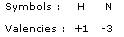So, chemical formula of ammonia is NH3.

Solution 13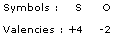Chemical formula of sulphur dioxide is SO2.

Solution 14

According to question-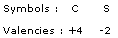Name and formula of the resulting compound is Carbon disulphide; CS2.

Solution 15

As the valency of element X is 4 and that of Y is 1, so the resulting formula is XY4.

Solution 16

When the valency shown by B is 4, then-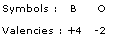The resulting compound is BO2.

When the valency shown by B is 6, then-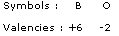The resulting compound is BO3.

Solution 17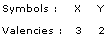Thus, the resulting compound is X2Y3.

Solution 18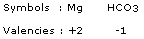Thus, the resulting compound is Mg(HCO3)2

Solution 19

(a). Bromide of element-

As valency of bromine is -1 and that of element X is +2 so, the resulting compound is XBr2.

(b). Oxide of element-

As valency of oxygen is -2 and that of element is +2 so, the resulting compound is XO.

Solution 20

(a). Sodium oxide-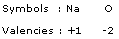Thus, the formula of sodium oxide is Na2O.

(b). Calcium carbonate-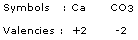Thus, the resulting compound is CaCO3.

Solution 21

(a).Molecular mass of Na2O = (2 x Na) + (1 x O) = (2 x 23) + (1 x 16) = 62u

(b). Molecular Mass of Al2O3 = (2 x Al) + (3 x O) = (2 x 27) + (3 x 16) = 102u

Solution 23

(a). CH3COONa : Na+ (cation) and CH3COO- (anion)

(b). NaCl : Na+ (cation) and Cl- (anion)

(c). H2 : It is a covalent molecule. So, cation and anion are not present.

(d). NH4NO3 : NH4+ (cation) and NO3- (anion)

Solution 24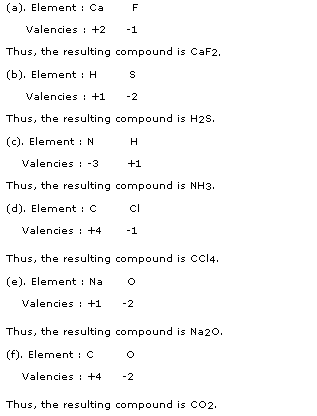Solution 25

i. Ionic compounds- The compounds which are formed by combination of metals and non-metals are called ionic compounds. For ex: CaCl2 and CaCO3.

ii. Molecular compounds- These compounds are formed by the combination between two non-metal elements. For ex. HCl and H2S.

## Chapter 3 - Atoms and Molecules 152

Solution 27

(a)A cation is formed by the loss of one or more electrons by an atom. For ex. Magnesium loses 2 electron to form Mg+2.

An anion is formed by the gain of one or more electrons by an atom. For Ex. Chlorine loses one electron to form Cl-.

(b) (i). Na2S

(ii). Cu (NO3)2

Solution 28

(i).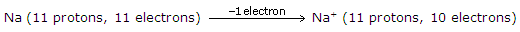The reason for positive charge on sodium is the loss of electron.

(ii).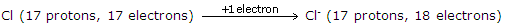The reason for negative charge on chlorine is the gain of electron.

Solution 29

(a). Simple ions: Br- and Na+ ; Compound ions: NH4+ and Al+3

(b). (i). YCl4 (ii). YO2  (iii). Y (SO4)2  (iv). Y (CO3)2  (v). Y (NO3)4

Solution 30

(a). The simplest combination of ions that produces an electrically neutral unit, is called 'formula unit' of the ionic compound.

Formula unit of sodium chloride - NaCl

Formula unit of magnesium chloride - MgCl2

(b).

(i). Formula Mass of Calcium chloride (CaCl2) = 1xCa + 2xCl = (40+71) u = 111 u

(ii). Formula Mass of Sodium carbonate (Na2CO3) = 2xNa + 1xC + 3xO= (2x23 + 1x12 + 3x16) u = 106 u

## Chapter 3 - Atoms and Molecules 153

Solution 46

(a). Let the valency of element A be y, then

2y + 5(-2) = 0

So, y = valency of element A = 5

(b). As valency of element A is 5 and valency of chlorine is -1,

So, the formula of chloride of A is ACl5.

Solution 47

Valency of X -

(i). In H2X : -2

(ii).In CX2 : -2

(iii).In XO2 : +4

(iv).In XO3 : +6

Solution 48

Let the valency of X be y, then

2 x (+3) + 3 x y = 0

So, valency of X = y = -2

As valency of Mg is +2 and that of X is -2 so the formula of Magnesium salt of X will be MgX.

Solution 49

According to formula M2CO3, valency of M is +1.

(a). formula of iodide = MI (as valency of iodine is -1)

(b). formula of nitride = M3N (as valency of nitrogen is -3)

(c). formula of phosphate = M3PO4

Solution 50

(a). Anion will be formed by element X ; Symbol : X-

(b). (i). No. of protons in X = 17

(ii). No. of electrons in X = 18

(iii). No. of neutrons in X = 18

(c). Cation will be formed by element Y ; Symbol : Y+

(d). (i). No. of protons in Y = 11

(ii). No. of electrons in Y = 10

(iii). No. of neutrons in Y = 12

(e).Atomic mass of X = No. of protons(17) + No. of neutrons(18) = 35 u

Atomic mass of Y = No. of protons (11) + No. of neutrons (12) = 23 u

(f). Element X is Chlorine (Cl).

Element Y is Sodium (Na).

## Chapter 3 - Atoms and Molecules 172

Solution 2

1 mole

Solution 3

6.022 x 1023

Solution 4

One mole of atoms (6.022 x 1023 atoms)

Solution 5

6.022 x 1023

Solution 6

Solution 7

Given mass of oxygen = 12g

Molar mass of oxygen = 32g

No. of moles = Given mass / Molar mass = 12g /32g = 0.375

Solution 8

No. of moles = 3.6g / 18g = 0.2 mole

Solution 9

Mass of 0.2 moles of oxygen atoms = 0.2 x 16 = 3.2g

Solution 10

Mass of 2 moles of nitrogen atoms = 2 x 14 = 28g

Solution 11

(a). 6.022 x 1023

(c). 60/12 = 5

(d). 0.5 x 40 g = 20 g

(e). 64g/16g = 4

Solution 12

(a). 6.022 x 1023 atoms

(b). One Mole

Solution 13

One mole of O2 = 32 gm

6.022 x 1023 molecules of O2 have mass = 32 gm

So, 12.044 x 10 25 molecules of O2 will have mass = 6400 gm = 6.4 Kg

Solution 14

One mole of ammonia contains = 6.022 x 1023 molecules of ammonia.

So, 1.5 moles of ammonia contains = 1.5 x 6.022 x 1023 molecules

= 9.033 x 1023 molecules of ammonia.

Solution 15

Given mass of CaCO3 = 10g

Molar mass of CaCO3 = 1xCa + 1xC + 3xO = (40+12+48)gm = 100gm

So, no. of moles of CaCO3 = Given mass/Molar mass = 10/100 = 0.1 moles

Solution 16

One mole of O2 contains = 6.022 x 1023 molecules of oxygen

So, 1 molecule of O2 has = 1/6.022 x 1023 moles of O2

Therefore, 1.2 x 1022 molecules of O2 will have = 1.2 x 1022 / 6.022 x 1023 moles of O2

= 0.0199 moles of O2

Solution 17

6.022 x 1023 molecules of N2 weigh = 28 gm

So, 1 molecule of N2 will weigh = 28 / 6.022 x 1023 grams of N2

= 4.648 x 10-23 grams of N2

Solution 18

1 mole of sodium weighs = 23 gm

So, 1 gm of sodium will have = 1/23 moles of sodium

Therefore, 34.5 gm of sodium will have = 34.5/23 = 1.5 moles of sodium.

Solution 19

1 mole of Zn = 65gm of zinc = 6.022 x 1023 atoms of zinc

Given mass of zinc = 10gm

No. of moles of zinc = 10/65 = 0.15 moles of zinc

Total no. of atoms in 0.15 moles = 0.15 x 6.022 x 1023 atoms of Zn

= 9.264 x 1022 atoms of Zn

Solution 20

Mass of 6.022 x 1023 atoms of Carbon = 12 g

So, Mass of 1 Carbon atom = 12/6.022 x 1023 g

Hence, mass of 3.011 x 1024 atoms of Carbon = 3.011 x 1024 x 12 / 6.022x 1023 = 60 g

Solution 21

6.022 x 1023 atoms of Oxygen weigh = 16 g

So, mass of 1 atom of Oxygen = 16/6.022 x 1023 = 2.656 x 10-23 g.

## Chapter 3 - Atoms and Molecules 173

Solution 22

1 mole of hydrogen has = 6.022 x 1023 atoms of hydrogen

So, 0.25 moles of hydrogen will have = 6.022 x 1023 x 0.25 = 1.50 x 1023 atoms of hydrogen.

Solution 24

Given mass of CHCl3 = 0.0239 g

Molar mass of CHCl3 = 1 x C + 1 x H + 3 x Cl = 119.5 g

No. of moles = Given mass/ Molar mass

No. of moles = 0.0239/119.5 = 0.0002

So, no. of molecules present in 0.0239 g of chloroform = 0.0002 x 6.022 x 1023

= 12.044 x 1019 molecules

Solution 29

Molar mass of CO2 = 44g

Molar mass of H2O = 18g

Mass of 5 mole of H2O = 5 x 18g = 90 g

Mass of 5 mole of CO2 = 5 x 44g = 220 g

So, 5 mole of H2O and 5 mole of CO2 do not have same mass.

And the difference in their masses = 220 g - 90 g = 130 g

Solution 31

(a). A group of 6.022 x 1023 particles (atoms, molecules or ions) of a substance is called a mole of that substance. One mole represents the amount of a substance equal to its 'GRAM ATOMIC MASS' or 'GRAM MOLECULAR MASS' and 6.022 x 1023 no. of particles of the substance.

(b). 1.5 moles of Na2SO3 has 3 moles of Na, 1.5 mole of S and 4.5 moles of O.

Thus, mass of sodium = 3 x 23 g = 69g

Mass of sulphur = 1.5 x 32 = 48g

Mass of oxygen = 4.5 x 16 g = 72g

Solution 32

(a) A mole of carbon atoms means a carbon sample weighing 12 g and containing 6.022 x 1023 carbon atoms.

(b) 1 mole of aluminium weighing 27g has = 6.022 x 1023 atoms of Al

So, 1g of Al has = 0.22 x 1023 atoms of Al

Hence, 50g of Al will have = 50 x 0.22 x 1023 atoms of Al

= 11 x 1023 atoms of Al

1 mole of iron weighing 56g has = 6.022 x 1023 atoms of Fe

So, 1g of Fe has = 0.10 x 1023 atoms of Fe

Hence, 50g of Fe will have = 50 x 0.10 x 1023 atoms of Fe = 5 x 1023 atoms of Fe

Thus, 50g of Al has more no. of atoms as compared to 50g of Fe.

Solution 33

(a). The amount of substance whose mass in grams is numerically equal to its atomic mass, is called gram atomic mass of that substance.

Gram atomic mass of oxygen is 16g.

(b). Moles of oxygen atom are -

(i). Al2O3 : 3 mole

(ii). CO2 : 2 mole

(iii).Cl2O7 :  7 mole

(iv). H2SO4 : 4 mole

(v). Al2(SO4)3 : 12 mole

Solution 34

(a). The amount of substance whose mass in grams is numerically equal to its molecular mass is called gram molecular mass of that substance.

Gram molecular mass of the oxygen is 32g.

(b). Given mass of sulphur = 100g

Molar mass of S8 = 32 x 8 g = 256g

No. of moles = Given mass / Molar mass = 100/ 256 = 0.39 moles.

Solution 35

(a). The molar mass of the substance is the mass of 1 mole of that substance. Molar mass is generally expressed in grams or 'g'.

(b).

(i). Molar mass of ozone (O3) = 3 x gram atomic mass of O = 3 x 16 g = 48 g/ mole

(ii). Molar mass of Ethanoic acid (CH3COOH) = 2xC + 4xH + 2xO

= (24 + 4 + 32)u = 60 g/ mole

Solution 26

32 g of oxygen (1 mole of oxygen) has = 6.022 x 1023 molecules of oxygen

So, 4 g of oxygen will have = 6.022 x 1023  x 4/32 = 7.528 x 1022 molecules of oxygen.

Solution 30

240g of calcium has = 240/40 = 6 moles

240g of magnesium has = 240/24 = 10 moles

So, required mole ratio = 6:10 = 3:5

Solution 25

1 mole of Na2CO3 = 106g

So, 5 x mole of Na2CO3 = 5 x 106g = 530g

Solution 28

1 mole of H2S weighs = 34 g

So, 0.17 mole of H2S will weigh = 34 x 0.17 g = 5.78 g

Solution 27

Molar mass of glucose = 180 g

180 g of glucose has = 1 mol

So, 100 g of glucose will have = 1 x 100/180 = 0.55 moles

## Chapter 3 - Atoms and Molecules 174

Solution 43

1 mole of SO2 = Mass of S + 2 x Mass of O = 64 grams

64 g of SO2 = 1 mole

So, 1 g of SO2 = 1/64 mole

Now since equal moles of all the substances contain equal number of molecules so, 1/64 mole of O2 will also contain x molecules like SO2.

32 g of oxygen = 1 mole

So, 1 g of oxygen = 1/32 mole

Now, 1/64 mole of oxygen contains = x molecules

So, 1/32 mole of oxygen will contain = x x 64/32 = 2x molecules

Solution 44

Mass of one molecule of substance = 4.65 x 10-23 u

So, mass of 1 mole of substance = Mass of 6.022 x 1023 molecules of the substance

= 4.65 x 10-23 x 6.022 x 1023 u = 28 u

The substance is Nitrogen with molecular mass 28 u.

Solution 45

Molar mass of SO2 = (32 + 2x16) g = 64g

Molar mass of oxygen (O2) = 32g

Given mass of SO2 = 10g = Given mass of oxygen (O2)

1 mole of substance = 6.023 x 1023 particles of the substance

(a). No. of moles of SO2 = 10g/64g = 0.15

Total no. of molecules of SO2 = 0.15 x 6.022 x 1023 = 0.90 x 1023 molecules of SO2

(b). No. of moles of O2 = 10g/32g = 0.31

Total no. of molecules of O2 = 0.31 x 6.022 x1023 = 1.88 x 1023 molecules of O2

Thus, 10g of O2 contains more no. of molecules.

Solution 46

Given mass of nitrogen = 56g

Molar mass of nitrogen = 14g

No. of moles of nitrogen = 56g/14g = 4 moles

Equal number of moles of all the susbtances contain equal number of molecules.

So, 4 moles of nitrogen and 4 moles of oxygen contains same no. of molecules.

Hence, mass of 4 mole of oxygen = 4 x 16g = 64 g

Solution 47

Given mass of water = 1.8g

Molar mass of water = 18g

No. of moles of water = 1.8g/18g = 0.1 moles

Equal number of moles of all the susbtances contain equal number of molecules.

So, 0.1 moles of water and 0.1 moles of nitrogen contains same no. of molecules.

Hence, mass of 0.1 mole of nitrogen = 0.1 x 28 g = 2.8 g

Solution 48

32 g of S = 1 mole

So, 1 g of S = 1/32 mole

Now since equal moles of all the substances contain equal number of atoms so, 1/32 mole of oxygen will also contain x atoms like S.

16 g of oxygen = 1 mole

So, 1 g of oxygen = 1/16 mole

Now, 1/32 mole of oxygen contains = x atoms

So, 1/16 mole of oxygen will contain = x x 32/16 = 2x atoms

Solution 49

Given mass of carbon = 6g

Molar mass of carbon = 12g

No. of moles of carbon = 6g/12g = 0.5 moles

Equal number of moles of all the susbtances contain equal number of molecules.

So, 0.5 moles of carbon and 0.5 moles of magnesium contains same no. of molecules.

Hence, mass of 0.5 mole of magnesium = 0.5 x 24g = 12g

Solution 50

(i). Mass of 1 g of element X = 2 x 10 -23 g

Mass of 1 mole of element X = 2 x 10-23 x 6.022 x 1023 = 12.044 g

Molar mass of the element X = mass of 1 mole of element = 12 u

(ii). Element X is CARBON.

### STUDY RESOURCES

REGISTERED OFFICE : First Floor, Empire Complex, 414 Senapati Bapat Marg, Lower Parel, Mumbai - 400013, Maharashtra India.